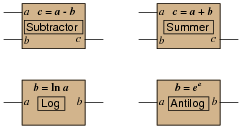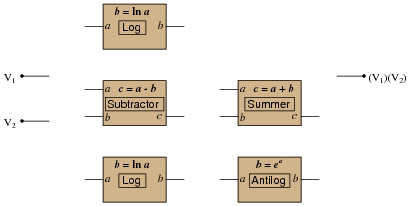# Logarithms for Analog Circuits

• #### Question 1

The concept of a mathematical power is familiar to most students of algebra. For instance, ten to the third power means this:

 103 = 10 ×10 ×10 = 1000

. . . and eight to the seventh power means this:

 87 = 8 ×8 ×8 ×8 ×8 ×8 ×8 = 2,097,152

Just as subtraction is the inverse function of addition, and division is the inverse function of multiplication (because with inverse functions, one “undoes” the other), there is also an inverse function for a power and we call it the logarithm.

Re-write the expression 103 = 1000 so that it uses the same quantities (10, 3, and 1000) in the context of a logarithm instead of a power, just as the subtraction is shown here to be the inverse of addition, and division is shown to be the inverse of multiplication in the following examples:

 3 + 8 = 11 ( and − are inverse functions) 11 − 3 = 8

 2 × 7 = 14 (× and ÷are inverse functions) 14 ÷ 2 = 7

 103 = 1000 (powers and logs are inverse functions) log10 ??? = ???

• #### Question 2

Given the following mathematical expression, write another one defining a logarithm using the same variables:

 If: xy = z Then: log? ? = ?

• #### Question 3

Electronic calculators with logarithm capability have at least two different types of logarithms: common logarithm and natural logarithm, symbolized as “log” and “ln”, respectively. Explain what the difference is between these two types of logarithms.

• #### Question 4

Note the following logarithmic identities, using the “common” (base 10) logarithm:

 log10 = 1

 log100 = 2

 log1000 = 3

 log10000 = 4

In the first equation, the numbers 10 and 1 were related together by the log function. In the second equation, the numbers 100 and 2 were related together by the same log function, and so on.

Rewrite the four equations together in such a way that the same numbers are related to each other, but without writing “log”. In other words, represent the same mathematical relationships using some mathematical function other than the common logarithm function.

• #### Question 5

Note the following logarithmic identities, using the “common” (base 10) logarithm:

 log0.1 = −1

 log0.01 = −2

 log0.001 = −3

 log0.0001 = −4

In the first equation, the numbers 0.1 and 1 were related together by the log function. In the second equation, the numbers 0.01 and 2 were related together by the same log function, and so on.

Rewrite the four equations together in such a way that the same numbers are related to each other, but without writing “log”. In other words, represent the same mathematical relationships using some mathematical function other than the common logarithm function.

• #### Question 6

Examine the following progression of mathematical statements:

 (102)(103) = 100000

 102 + 3 = 100000

 105 = 100000

What does this pattern indicate? What principle of algebra is illustrated by these three equations?

Next, examine this progression of mathematical statements:

 log105 = log100000 = 5

 log102 + 3 = log100000 = 5

 log102 + log103 = log100000 = 5

What does this pattern indicate? What principle of algebra is illustrated by these three equations?

• #### Question 7

Examine this progression of mathematical statements:

 (100)(1000) = 100000

 (100)(1000) = 105

 log[(100)(1000)] = log105

 log100 + log1000 = log105

 log102 + log103 = log105

 2 + 3 = 5

What began as a multiplication problem ended up as an addition problem, through the application of logarithms. What does this tell you about the utility of logarithms as an arithmetic tool?

• #### Question 8

Suppose you owned a scientific calculator with two broken buttons: the multiply (×) and divide (÷). Demonstrate how you could solve this simple multiplication problem using only logarithms, addition, and antilogarithms (powers):

 7 ×5 = ???

The answer to this problem was easy enough for you to figure out without a calculator at all, so here are some more practice problems for you to try:

23 ×35 =
781 ×92 =
19.4 ×60 =
0.019 ×2.6 =
• #### Question 9

Examine this progression of mathematical statements:

 1000 100 = 10

 1000 100 = 101

 log ( 1000 100 ) = log101

 log1000 − log100 = log101

 log103 − log102 = log101

 3 − 2 = 1

What began as a division problem ended up as a subtraction problem, through the application of logarithms. What does this tell you about the utility of logarithms as an arithmetic tool?

• #### Question 10

Suppose you owned a scientific calculator with two broken buttons: the multiply (×) and divide (÷). Demonstrate how you could solve this simple multiplication problem using only logarithms, addition, and antilogarithms (powers):

 12 ÷3 = ???

The answer to this problem was easy enough for you to figure out without a calculator at all, so here are some more practice problems for you to try:

122 ÷35 =
781 ÷92 =
19.4 ÷60 =
3.5 ÷0.21 =
• #### Question 11

Examine this progression of mathematical statements:

 (1000)2 = 1000000

 (1000)2 = 106

 log[(1000)2] = log106

 (2)(log1000) = log106

 (2)(log103) = log106

 (2)(3) = 6

What began as an exponential problem ended up as a multiplication problem, through the application of logarithms. What does this tell you about the utility of logarithms as an arithmetic tool?

• #### Question 12

Suppose you owned a scientific calculator with two broken buttons: the power (yx) and root (x

√{y}). Demonstrate how you could solve this simple power problem using only logarithms, multiplication, and antilogarithms (powers):

 34 = ???

The answer to this problem was easy enough for you to figure out without a calculator at all, so here are some more practice problems for you to try:

256 =
5643 =
0.2242 =
410.3 =
• #### Question 13

Examine this progression of mathematical statements:

 √ 1000 = 101.5

 log √ 1000 = log( 101.5 )

 log( 10001/2 ) = log( 101.5 )

 1 2 (log1000) = log( 101.5 )

 1 2 (log103) = log( 101.5 )

 3 2 (log10) = log( 101.5 )

 3 2 (1) = log( 101.5 )

 3 2 = log( 101.5 )

 3 2 = 1.5

What began as a fractional exponent problem ended up as a simple fraction, through the application of logarithms. What does this tell you about the utility of logarithms as an arithmetic tool?

• #### Question 14

Suppose you owned a scientific calculator with two broken buttons: the power (yx) and root (x

√{y}). Demonstrate how you could solve this simple root problem using only logarithms, division, and antilogarithms (powers):

 3 √ 8 = ???

The answer to this problem was easy enough for you to figure out without a calculator at all, so here are some more practice problems for you to try:

4 √{13} =
5 √{209} =
2.5 √{9935} =
9.2 √{0.15} =
• #### Question 15

You may be wondering why anyone would bother using logarithms to solve arithmetic problems for which we have perfectly good and effective digital electronic calculator functions at our disposal. For example, why would anyone do this:

 10log7 + log5

. . . when they could just do the following on the same calculator?

 7 ×5

The quick answer to this very good question is, “when it is more difficult to directly multiply two numbers.” The trouble is, most people have a difficult time imagining when it would ever be easier to take two logarithms, add them together, and raise ten to that power than it would be to simply multiply the original two numbers together.

The answer to that mystery is found in operational amplifier circuitry. As it turns out, it is much easier to build single opamp circuits that add, subtract, exponentiate, or take logarithms than it is to build one that directly multiplies or divides two quantities (analog voltages) together.

We may think of these opamp functions as “blocks” which may be interconnected to perform composite arithmetic functions:Using this model of specific math-function “blocks,” show how the following set of analog math function blocks may be connected together to multiply two analog voltages together:• #### Question 16

Logarithms have interesting properties, which we may exploit in electronic circuits to perform certain complex operations. In this question, I recommend you use a hand calculator to explore these properties.

Calculate the following:

10log3 =
log(108) =
eln3 =
ln(e8) =
10(log3 + log5) =
e(ln3 + ln5) =
10(log2.2 + log4) =
e(ln2.2 + ln4) =
10(log12 − log4) =
e(ln12 − ln4) =
10(2 log3) =
e(2 ln3) =
10([log25/2]) =
e([ln25/2]) =